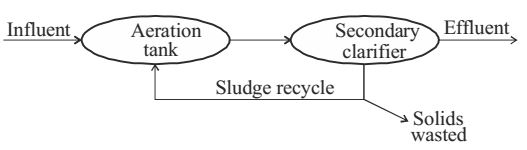#### Municipal Solid Waste

Direction: An activated sludge system (sketched below) is operating at equilibrium with the following information. Waste water related data: flow rate = 500 m³/hour, influent BOD = 150 mg/l, effluent BOD = 10 mg/l. Aeration tank related data: hydraulic retention time = 8 hours, mean-cell-residence time = 240 hours, volume = 4000 m³, mixed liquor suspended solids = 2000 mg/l.1. The mass (in kg/day) of solids wasted from the system is
1. 24000
2. 1000
3. 800
4. 33
##### Correct Option: C

 Sludge age (Qc) = Mass of biomass in system Mass of biomass leaving the system

Therefore,
 Mass of biomass leaving system = Mass of biomass in system Qc

 = Volume × MLSS Qc

 = 4000 × 10-3 2000 × 10-6 240

 = 8000 kg/l = 800 kg/day. 240